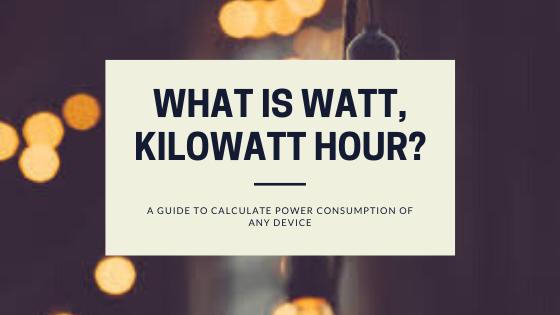# What is Watt (W), Kilowatt (KW), Kilowatt hour (kWh) (unit) of electricity?Share the article

Watt, kilowatt, Kilowatt hour are the three electrical terms that most people find confusing. In this article we will discuss about what is watt (W), what is kilowatt (kW) and what is kilowatt hour (kWh) of electricity or unit of electricity.

Watt is the unit of power. Watt and Kilowatt shows the rate at which power is consumed by a device. For example a 100 watt TV will consumes power at a rate of 100 watt per hour.

Kilowatt is a bigger unit of power used to depict bigger watt of power (just like kilometer is to meter). 1 kilowatt = 1000 watt.

Kilowatt hour is the actual energy consumed by the device in an hour. For example a 100 watt TV running for 24 hours consumes 100 watt x 24 hours = 2400 watt hour or 2.4 kilowatt hour of electricity a.k.a 2.4 units of electricity.

## What is Watt (W)?

Watt is the unit of power. It means the rate at which electricity is consumed or produced by a device.

For example a 50 watt electric fan consumes power at a rate of 50 watts per hour, it does not means that electric fan consumed 50 units of electricity, it means it will consume power at a rate of 50 watts every hour.

Most appliances have a sticker which has their wattage written on it. Just like this.

The unit power is named as watt in respect of Scottish Inventor James Watt for his crucial role in transforming our world from one based on agriculture to one based on engineering and technology.

## What is Kilowatt (KW)?

Kilowatt is also a unit of power. Kilowatt (KW) is used for large devices that consume more power. For example a 1.5 kW air conditioner, 6 kW elevator. There are other bigger units like Megawatt (MW), Gigawatt (GW) to describe large power consumption or generation. For example 100 Megawatt (MW) coal power plant.

### Simple conversion between watt, kilowatt, megawatt, gigawatt :

• 1 Kilowatt (KW) = 1000 Watt (W)
• 1 Megawatt (MW) = 1000 Kilowatt (KW)
• 1 Gigawatt (GW) = 1000 Megawatt (MW)
• 1 Terawatt (TW) = 1000 Gigawatt (GW)
• 1 Petawatt (PW) = 1000 Terawatt (TW)

## What is Kilowatt Hour (kWh) or Unit of Electricity?

Units as described in electricity bill a.k.a kilowatt hour is the energy consumption of a device. For example a 50 watt electric fan running for 50 hours will consume 50 watt x 50 hours = 2500 watt hours = 2.5 kilowatt hours of electricity = 2.5 units of electricity.

1 kWh of electricity = 1 unit of electricity

### Simple conversion from watt hour to kilowatt hour to megawatt hour to gigawatt hour.

• 1 Kilowatt hour (kWh) = 1000 Watt hour (Wh).
• 1 Megawatt hour (MWH) = 1000 Kilowatt hour (kWh).
• 1 Gigawatt hour (GWH) = 1000 Megawatt hour (MWH).

If you need any more clarification on this topic then watch this video from The Engineering Mindset on What is kWh?

### What Can You Do With A Unit of Electricity?

#### How to calculate power consumption of any appliance:

To calculate power consumption of any device you can use the following equation,

Power consumed by a device (KWH) = Rated power (kW) x Operational hours (hrs)

For example power consumed by a,

• 100 watt television running for 10 hours = 100 watt x 10 hours = 1000 watt hours = 1 kWh
• 50 watt electric fan running for 30 hours = 50 watt x 30 hours = 1.5 kWh
• 1000 watt water pump running for 2 hours = 1000 watt x 2 hours = 2 kWh

This calculation does not work for devices like air conditioner, refrigerator which has a compressor which turns on and off as per requirement (duty cycle), here calculation is different.

For example a 2 kW air conditioner running for 4 hours won’t consume 8 kWh of electricity because the compressor which consumes the maximum power in an air conditioner is not running continuously for 4 hours, in fact it turns on and off as per the requirement of cooling space.

Use the below calculator to calculate power consumption of any device.

You can check these post to learn to calculate power consumption of other devices with example:

#### How to calculate amount of electricity bill you will get by using an appliance:

So far we know how to calculate units of electricity (kWh) consumed by any appliance. Now we can use this information to calculate the amount of electricity bill (your currency \$, Rs) you will get for using this appliance.

Amount of electricity bill for using an appliance (Rs) = Units consumed by an appliance (kWh) x Electricity tariff (Rs)

Tariff is the amount your electricity provider charges you for one unit of electricity. Tariff is measured in Rs / kWh.

So if we use a 100 TV for 24 hrs and Tariff is Rs 10 / kWh then,

Amount of electricity bill for using this 100 W TV for 24 hrs = 2.4 (kWh) x 10 (Rs/kWh)

= Rs 24.

## Conclusion on Watt, Kilowatt, Kilowatt hour:

Watt is the unit of power, it means the rate at which power is consumed by a device. Kilowatt hour (kWh) shows the actual electricity consumed by the device in an hour. A 50 Watt electric fan running for 100 hours consumes 5 kWh of electricity not 50 watt of electricity.

1 kilowatt = 1000 watt

1 kilowatt hour = 1 unit of electricity

### References:

You can check the following articles to know more about watt, kilowatt, kilowatt hour of electricity:

Share the article